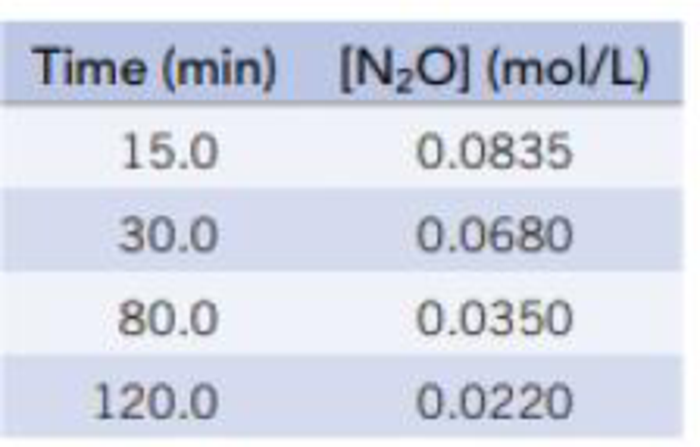# Data for the decomposition of dinitrogen monoxide N 2 O(g) → N 2 (g) + ½ O 2 (g) on a gold surface at 900 °C are given below. Verify that the reaction is first-order by preparing a graph of In[N 2 O] versus time. Derive the rate constant from the slope of the line in this graph. Using the rate law and value of k , determine the decomposition rate at 900 °C when [N 2 O] = 0.035 mol/L.### Chemistry & Chemical Reactivity

9th Edition
John C. Kotz + 3 others
Publisher: Cengage Learning
ISBN: 9781133949640

#### Solutions

Chapter
Section### Chemistry & Chemical Reactivity

9th Edition
John C. Kotz + 3 others
Publisher: Cengage Learning
ISBN: 9781133949640
Chapter 14, Problem 31PS
Textbook Problem
225 views

## Data for the decomposition of dinitrogen monoxideN2O(g) → N2(g) + ½ O2(g)on a gold surface at 900 °C are given below. Verify that the reaction is first-order by preparing a graph of In[N2O] versus time. Derive the rate constant from the slope of the line in this graph. Using the rate law and value of k, determine the decomposition rate at 900 °C when [N2O] = 0.035 mol/L.Interpretation Introduction

Interpretation: The rate of decomposition at 900°C has to be given.

Concept Introduction:

The rate of reaction is the quantity of formation of product or the quantity of reactant used per unit time.  The rate of reaction doesn’t depend on the sum of amount of reaction mixture used.

The raise in molar concentration of product of a reaction per unit time or decrease in molarity of reactant per unit time is called rate of reaction and is expressed in units of mol/(L.s).

Integrated rate law for first order reaction:

Consider A as substance, that gives the product based on the equation,

aAproducts

Where a= stoichiometric co-efficient of reactant A.

Consider the reaction has first-order rate law,

Rate=-Δ[A]Δt=k[A]

The integrated rate law equation can be given as,

ln[A]t[A]o=-kt

The above expression is called integrated rate law for first order reaction.

### Explanation of Solution

The reaction rate of the chemical reaction is calculated as,

Given:Time(min)ln[N2O](mol/L)15.02.48330.02.68880.03.352120.03.816Findthe slope for any two values:slope=Δln[N2O]Δt=2.688(2.482)3015=0.20515=0.0136k=slope=0.0136min1Therefore,[N2O]=0

### Still sussing out bartleby?

Check out a sample textbook solution.

See a sample solution

#### The Solution to Your Study Problems

Bartleby provides explanations to thousands of textbook problems written by our experts, many with advanced degrees!

Get Started

Find more solutions based on key concepts
Outline the foods you will eat (including quantities) that will provide the recommended RDA for calcium. List l...

Nutrition: Concepts and Controversies - Standalone book (MindTap Course List)

Define the following terms: a. chromosome b. chromatin

Human Heredity: Principles and Issues (MindTap Course List)

Why don’t red dwarfs become giant stars?

Horizons: Exploring the Universe (MindTap Course List)

In a game of American football. a quarterback takes the ball from the line of scrimmage. runs backward a distan...

Physics for Scientists and Engineers, Technology Update (No access codes included)

What were the greatest objections to Wegeners hypothesis?

Oceanography: An Invitation To Marine Science, Loose-leaf Versin